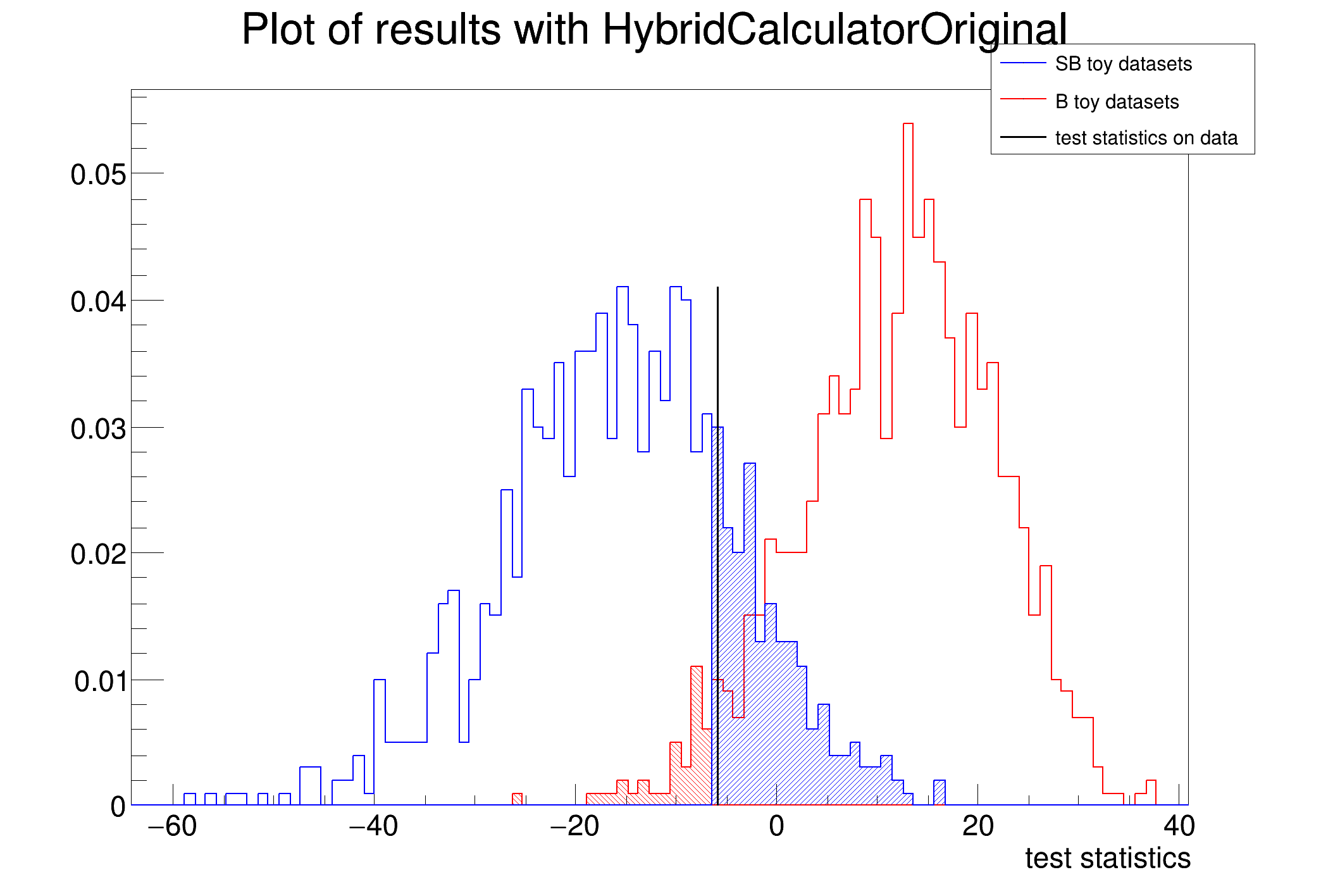ROOT   Reference GuideHybridOriginalDemo.C File Reference

## Detailed DescriptionExample on how to use the HybridCalculatorOriginal class

With this example, you should get: CL_sb = 0.130 and CL_b = 0.946 (if data had -2lnQ = -3.0742).␛[1mRooFit v3.60 -- Developed by Wouter Verkerke and David Kirkby␛[0m
Copyright (C) 2000-2013 NIKHEF, University of California & Stanford University
Test statistics has been evaluated for data
HybridCalculatorOriginal: run 1000 toy-MC experiments
with test statistics index: 1
marginalize nuisance parameters
....... toy number 0 / 1000
....... toy number 500 / 1000
Completed HybridCalculatorOriginal example:
- -2lnQ = -5.97789
- CL_sb = 0.194
- CL_b = 0.04
- CL_s = 4.85
- significance of data = 1.75069
- mean significance of toys = 2.65207
#include "RooRandom.h"
#include "RooRealVar.h"
#include "RooGaussian.h"
#include "RooPolynomial.h"
#include "RooArgSet.h"
#include "RooDataSet.h"
#include "RooExtendPdf.h"
#include "RooConstVar.h"
void HybridOriginalDemo(int ntoys = 1000)
{
using namespace RooFit;
using namespace RooStats;
// set RooFit random seed
/// build the models for background and signal+background
RooRealVar x("x", "", -3, 3);
RooArgList observables(x); // variables to be generated
// gaussian signal
RooGaussian sig_pdf("sig_pdf", "", x, RooConst(0.0), RooConst(0.8));
RooRealVar sig_yield("sig_yield", "", 20, 0, 300);
// flat background (extended PDF)
RooPolynomial bkg_pdf("bkg_pdf", "", x, RooConst(0));
RooRealVar bkg_yield("bkg_yield", "", 40, 0, 300);
RooExtendPdf bkg_ext_pdf("bkg_ext_pdf", "", bkg_pdf, bkg_yield);
// bkg_yield.setConstant(kTRUE);
sig_yield.setConstant(kTRUE);
// total sig+bkg (extended PDF)
RooAddPdf tot_pdf("tot_pdf", "", RooArgList(sig_pdf, bkg_pdf), RooArgList(sig_yield, bkg_yield));
// build the prior PDF on the parameters to be integrated
// gaussian contraint on the background yield ( N_B = 40 +/- 10 ie. 25% )
RooGaussian bkg_yield_prior("bkg_yield_prior", "", bkg_yield, RooConst(bkg_yield.getVal()), RooConst(10.));
RooArgSet nuisance_parameters(bkg_yield); // variables to be integrated
/// generate a data sample
RooDataSet *data = tot_pdf.generate(observables, RooFit::Extended());
// run HybridCalculator on those inputs
// use interface from HypoTest calculator by default
HybridCalculatorOriginal myHybridCalc(*data, tot_pdf, bkg_ext_pdf, &nuisance_parameters, &bkg_yield_prior);
// here I use the default test statistics: 2*lnQ (optional)
myHybridCalc.SetTestStatistic(1);
// myHybridCalc.SetTestStatistic(3); // profile likelihood ratio
myHybridCalc.SetNumberOfToys(ntoys);
myHybridCalc.UseNuisance(true);
// for speed up generation (do binned data)
myHybridCalc.SetGenerateBinned(false);
// calculate by running ntoys for the S+B and B hypothesis and retrieve the result
HybridResult *myHybridResult = myHybridCalc.GetHypoTest();
if (!myHybridResult) {
std::cerr << "\nError returned from Hypothesis test" << std::endl;
return;
}
/// nice plot of the results
HybridPlot *myHybridPlot =
myHybridResult->GetPlot("myHybridPlot", "Plot of results with HybridCalculatorOriginal", 100);
myHybridPlot->Draw();
/// recover and display the results
double clsb_data = myHybridResult->CLsplusb();
double clb_data = myHybridResult->CLb();
double cls_data = myHybridResult->CLs();
double data_significance = myHybridResult->Significance();
double min2lnQ_data = myHybridResult->GetTestStat_data();
/// compute the mean expected significance from toys
double mean_sb_toys_test_stat = myHybridPlot->GetSBmean();
myHybridResult->SetDataTestStatistics(mean_sb_toys_test_stat);
double toys_significance = myHybridResult->Significance();
std::cout << "Completed HybridCalculatorOriginal example:\n";
std::cout << " - -2lnQ = " << min2lnQ_data << endl;
std::cout << " - CL_sb = " << clsb_data << std::endl;
std::cout << " - CL_b = " << clb_data << std::endl;
std::cout << " - CL_s = " << cls_data << std::endl;
std::cout << " - significance of data = " << data_significance << std::endl;
std::cout << " - mean significance of toys = " << toys_significance << std::endl;
}
const Bool_t kTRUE
Definition: RtypesCore.h:89
RooAddPdf is an efficient implementation of a sum of PDFs of the form.
RooArgList is a container object that can hold multiple RooAbsArg objects.
Definition: RooArgList.h:21
RooArgSet is a container object that can hold multiple RooAbsArg objects.
Definition: RooArgSet.h:28
RooDataSet is a container class to hold unbinned data.
Definition: RooDataSet.h:33
RooExtendPdf is a wrapper around an existing PDF that adds a parameteric extended likelihood term to ...
Definition: RooExtendPdf.h:22
Plain Gaussian p.d.f.
Definition: RooGaussian.h:25
RooPolynomial implements a polynomial p.d.f of the form.
Definition: RooPolynomial.h:28
static TRandom * randomGenerator()
Return a pointer to a singleton random-number generator implementation.
Definition: RooRandom.cxx:53
RooRealVar represents a variable that can be changed from the outside.
Definition: RooRealVar.h:35
virtual void SetSeed(ULong_t seed=0)
Set the random generator seed.
Definition: TRandom.cxx:597
RooConstVar & RooConst(Double_t val)
RooCmdArg Extended(Bool_t flag=kTRUE)
Double_t x[n]
Definition: legend1.C:17
The namespace RooFit contains mostly switches that change the behaviour of functions of PDFs (or othe...
Namespace for the RooStats classes.
Definition: Asimov.h:19

Definition in file HybridOriginalDemo.C.